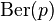# Bernoulli distribution facts for kids

Kids Encyclopedia Facts

The Bernoulli distribution is a probability distribution. It takes a value of 1 with probability p and a value of 0 with probability 1-p. It is sometimes written as$\operatorname{Ber}(p)$. It is used in probability theory and statistics. It is named after a Swiss scientist Jacob Bernoulli.

## Overview

A Bernoulli distribution is useful because it can be used to approximate the outcomes of an experiment (such as tossing a coin) as a range of percentages. It represents a "yes or no" type experiment. If the experiment succeeds, then it is given the value 1. If the experiment does not succeed, it is given value 0. This can be used, for example, in tossing a coin, where "1" means it lands on "heads", and "0" means it lands on "tails" (or the other way around).

This distribution has only one parameter, the probability of a success (p). In fact, Bernoulli distribution is the binomial distribution with n=1, and n independent and identically distributed Bernoulli distributions also give rise to binomial distribution.

## Related pagesIn Spanish: Distribución Bernoulli para niños